2

# Delete Node In BST

Difficulty: MEDIUM
Avg. time to solve
10 min
Success Rate
90%

Problem Statement
Suggest Edit

#### A binary search tree (BST) is a binary tree data structure which has the following properties.

``````• The left subtree of a node contains only nodes with data less than the node’s data.
• The right subtree of a node contains only nodes with data greater than the node’s data.
• Both the left and right subtrees must also be binary search trees.
``````
##### Example:
``````For the given BST, if we want to delete the node with data 5 :
``````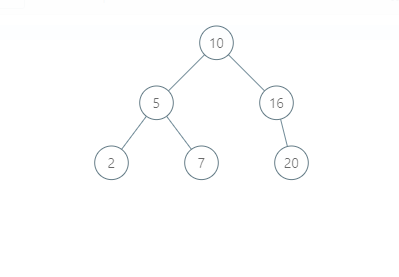``````The modified BST will be:
``````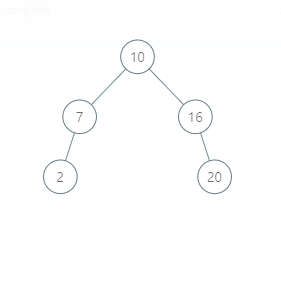``````Please notice that another valid answer is:
``````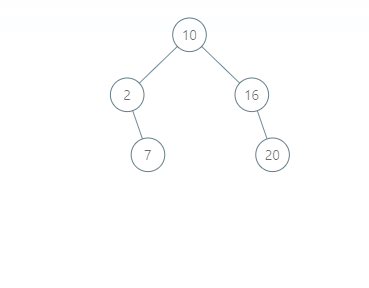#### Note :

``````1. The node which we want to delete will always be present in the given tree.

2. If after deletion the tree becomes empty, print -1.
``````
##### Input Format:
``````The first line contains an integer 'T' which denotes the number of test cases.

The first line of each test case contains the elements of the tree in the level order form separated by a single space. If any node does not have a left or right child, -1 is used in its place. Refer to the example given below.

The second line of each test case contains the data of the node which we want to delete.
``````
##### Example:
``````Elements are in the level order form. The input consists of values of nodes separated by a single space in a single line. In case a node is null, we take -1 in its place.

For example, the input for the tree depicted in the below image would be :
1 2 3 4 -1 5 6 -1 7 -1 -1 -1 -1 -1 -1
``````##### Explanation :
``````Level 1 :
The root node of the tree is 1

Level 2 :
Left child of 1 = 2
Right child of 1 = 3

Level 3 :
Left child of 2 = 4
Right child of 2 = null (-1)
Left child of 3 = 5
Right child of 3 = 6

Level 4 :
Left child of 4 = null (-1)
Right child of 4 = 7
Left child of 5 = null (-1)
Right child of 5 = null (-1)
Left child of 6 = null (-1)
Right child of 6 = null (-1)

Level 5 :
Left child of 7 = null (-1)
Right child of 7 = null (-1)

1
2 3
4 -1 5 6
-1 7 -1 -1 -1 -1
-1 -1
``````
##### Note :
`````` 1. The first not-null node (of the previous level) is treated as the parent of the first two nodes of the current level. The second not-null node (of the previous level) is treated as the parent node for the next two nodes of the current level and so on.

2. The input ends when all nodes at the last level are null (-1).
``````
##### Output Format :
``````For each test case, print the inorder traversal of the modified Binary Search Tree.
``````
##### Note:
``````1. The inorder traversal of a binary tree is the traversal method in which for any node its left subtree is visited first, then the node itself, and then the right subtree.

2. You don't need to print the output, it has already been taken care of. Just implement the given function.
``````
##### Constraint :
``````1 <= T <= 100
1 <= N <= 5000
0 <= data <= 10^5

Where ‘N’ is the number of nodes in the binary search tree and ‘data' denotes data contained in the node of the binary search tree.

Time limit: 1 sec
``````
##### Sample Input 1:
``````1
20 -1 -1
20
15 10 20 8 12 16 25 -1 -1 -1 -1 -1 -1 -1 -1
10
``````
##### Sample output 1:
``````-1
8 12 15 16 20 25
``````
##### Explanation of Sample output 1:
``````In test case 1, there is only one node with the value 20, thus after deleting it the tree would be empty.

In test case 2, the tree can be represented as follows:
``````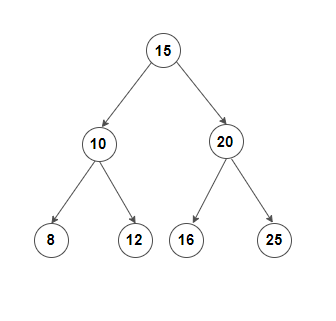``````After the deletion of the node with data 10 the BST will be:
``````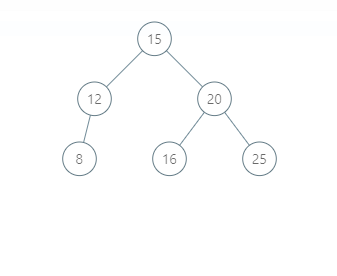``````The inorder traversal of this modified BST is 8 12 15 16 20 25.

Another valid modified BST can be:
``````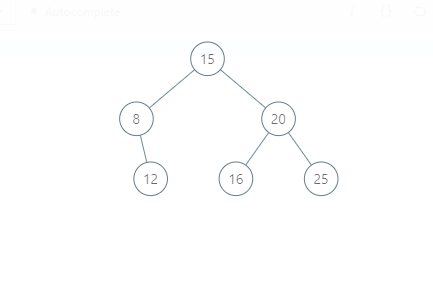``````The inorder traversal of this modified BST is also 8 12 15 16 20 25.
``````
##### Sample Input 2:
``````2
10 5 -1 -1 -1
5
6 4 -1 -1 5
6
``````
##### Sample output 2:
``````10
4 5
``````
##### Explanation of Sample Output 2:
``````In test case 1, there are only two nodes in the Binary Search Tree and we are deleting the node with value 5. Thus the only node left will the node with value 10.

In test case 2, the tree can be represented as follows:
``````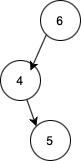``````After the deletion of the node with data 6 the BST will be:
``````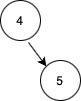``````The inorder traversal of this modified BST is 4 5.
``````Console